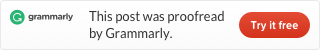Categories

# LeetCode 973 K Closest Points to Origin [sort] java

We have a list of `points` on the plane.  Find the `K` closest points to the origin `(0, 0)`.

(Here, the distance between two points on a plane is the Euclidean distance.)

You may return the answer in any order.  The answer is guaranteed to be unique (except for the order that it is in.)

Example 1:

```Input: points = [[1,3],[-2,2]], K = 1
Output: [[-2,2]]
Explanation:
The distance between (1, 3) and the origin is sqrt(10).
The distance between (-2, 2) and the origin is sqrt(8).
Since sqrt(8) < sqrt(10), (-2, 2) is closer to the origin.
We only want the closest K = 1 points from the origin, so the answer is just [[-2,2]].
```

Example 2:

```Input: points = [[3,3],[5,-1],[-2,4]], K = 2
Output: [[3,3],[-2,4]]
(The answer [[-2,4],[3,3]] would also be accepted.)
```

Note:

1. `1 <= K <= points.length <= 10000`
2. `-10000 < points[i] < 10000`
3. `-10000 < points[i] < 10000`

I make a class distance, include the distance d and the index. First, we calculate all distances, put them into a distance array. Then we sort the array by distance.

`Running time is 36 ms faster than 46.32% Java submissions.`